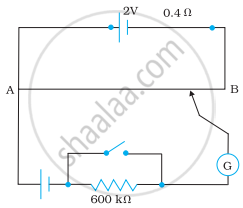# Figure shows a potentiometer with a cell of 2.0 V and internal resistance 0.40 Ω maintaining a potential drop across the resistor wire AB. - Physics

Numerical

Figure shows a potentiometer with a cell of 2.0 V and internal resistance 0.40 Ω maintaining a potential drop across the resistor wire AB. A standard cell which maintains a constant emf of 1.02 V (for very moderate currents up to a few mA) gives a balance point at 67.3 cm length of the wire. To ensure very low currents drawn from the standard cell, very high resistance of 600 kΩ is put in series with it, which is shorted close to the balance point. The standard cell is then replaced by a cell of unknown emf ε and the balance point found similarly, turns out to be at 82.3 cm length of the wire.(a) What is the value ε?

(b) What purpose does the high resistance of 600 kΩ have?

(c) Is the balance point affected by this high resistance?

(d) Is the balance point affected by the internal resistance of the driver cell?

(e) Would the method work in the above situation if the driver cell of the potentiometer had an emf of 1.0 V instead of 2.0 V?

(f) Would the circuit work well for determining an extremely small emf, say of the order of a few mV (such as the typical emf of a thermo-couple)? If not, how will you modify the circuit?

#### Solution

(a) Constant emf of the given standard cell, E1 = 1.02 V

Balance point on the wire, l= 67.3 cm

A cell of unknown emf, ε, replaced the standard cell. Therefore, new balance point on the wire, l = 82.3 cm

The relation connecting emf and balance point is,

"E"_1/"l"_1 = ε/"l"

ε = "l"/"l"_1 xx "E"_1

= 82.3/67.3 xx 1.02

= 1.247 V

The value of unknown emf is 1.247 V.

(b) The purpose of using the high resistance of 600 kΩ is to reduce the current through the galvanometer when the movable contact is far from the balance point.

(c) The balance point is not affected by the presence of high resistance.

(d) The point is not affected by the internal resistance of the driver cell.

(e) The method would not work if the driver cell of the potentiometer had an emf of 1.0 V instead of 2.0 V. This is because if the emf of the driver cell of the potentiometer is less than the emf of the other cell, then there would be no balance point on the wire.

(f) The circuit would not work well for determining an extremely small emf. As the circuit would be unstable, the balance point would be close to ending A. Hence, there would be a large percentage of errors.

The given circuit can be modified if a series resistance is connected with the wire AB. The potential drop across AB is slightly greater than the emf measured. The percentage error would be small.

Concept: Potentiometer
Is there an error in this question or solution?

#### APPEARS IN

NCERT Physics Part 1 and 2 Class 12
Chapter 3 Current Electricity
Exercise | Q 3.22 | Page 130
NCERT Class 12 Physics Textbook
Chapter 3 Current Electricity
Exercise | Q 22 | Page 130
Share